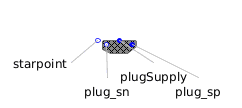Fixed Pressure - MapleSim Help

Fixed Pressure

Fixed pressure sourceDescription The Fixed Pressure component generates a constant pressure across its ports.Equations $p={p}_{A}-{p}_{B}=P$ $q={q}_{A}=-{q}_{B}$Variables

 Name Units Description Modelica ID $p$ $\mathrm{Pa}$ Pressure across component p $q$ $\frac{{m}^{3}}{s}$ Flow rate through component qConnections

 Name Description Modelica ID $\mathrm{portA}$ Upstream hydraulic port portA $\mathrm{portB}$ Downstream hydraulic port portBParameters

 Name Default Units Description Modelica ID $P$ $1$ $\mathrm{Pa}$ Pressure between port A and port B P